## Friday, May 29, 2009

### 404

404 = 22 x 101.

404 is a number that is the product of a prime and the square of a different prime.

The numbers 199989
394, 199989404 and 199989464 begin with the digits 394, 404, and 464 respectively.

404 has a representation as a sum of two squares: 404 = 202 + 22.

404 is the sum of seven positive fifth powers: 404 = 15 + 25 + 25 + 25 + 25 + 25 + 35.404 is the HTTP status code for "file not found," perhaps the most famous HTTP status code.

Source:
Number Gossip

## Thursday, May 28, 2009

### 317

"317 is a prime, not because we think so, or because our minds are shaped in one way rather than another, but because it is so, because mathematical reality is built that way." (G. H. Hardy, A Mathematician's Apology, 1940)

317 has a representation as a sum of two squares: 317 = 112 + 142.

317 is the hypotenuse of a primitive Pythagorean triple: 3172 = 752 + 3082.

The sum of the squares of the digits of the prime
317 is 59, another prime. Note that all odd digits are present.

317, 31, and 3 are prime numbers and so are 17 and 7.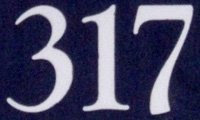Former pitching ace Walter "Big Train" Johnson once hurled a coin
317 feet across the Rappahannock River in Virginia.

Source:
Prime Curios!

## Wednesday, May 27, 2009

### 235

235 = 5 x 47.

235 has a unique representation as a sum of three squares: 235 = 12 + 32 + 152.

235 is the concatenation of the base 10 representations of the first three primes, and thus a Smarandache-Wellin number.

235 is the smallest number such that the set of its square's digits is a proper subset of the set of its own digits: 2352 = 55225 = (5 + 5 + 225)2.

235 is the smallest number whose first two digits are distinct primes such that their sum equals the third digit.On average, 19 solar years (228 solar months) is almost exactly
235 lunar months.

Source:
Number Gossip

## Tuesday, May 26, 2009

### 117

117 = 32 x 13.

117 has a representation as a sum of two squares: 117 = 62 + 92.

117 is the smallest possible value of the longest edge in an integer Heronian Tetrahedron. A Heronian tetrahedron, also called a perfect tetrahedron, is a (not necessarily regular) tetrahedron whose sides, face areas, and volume are all rational numbers.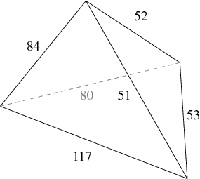1172 + 2 and 1172 – 2 are prime numbers.

117 can be written as the difference of prime squares (112 – 22) or prime cubes (53 – 23).

117 is 99 in base 12.The Lockheed F-117 Nighthawk is stealth ground attack military aircraft.

## Friday, May 22, 2009

### 43

43 is a prime number.

43 is the smallest prime formed from reverse concatenation of two consecutive numbers.

The first 43 digits of 43! form a prime number.

It is possible to form three sums of primes with the prime value of 43 using the ten first primes: 2 + 5 + 7 + 29 = 3 + 17 + 23 = 11 + 13 + 19 = 43.London was founded as the Roman town of Londinium in 43 A.D.

Source: Prime Curios!

## Thursday, May 21, 2009

### 990

990 = 2 x 32 x 5 x 11.

990 is a triangular number, the sum of the first 44 positive integers.

990 is the product of three consecutive integers: 9 x 10 x 11 = 990.

990 is the sum of six consecutive primes: 151 + 157 + 163 + 167 + 173 + 179 = 990.

A prime magic square with magic sum 990: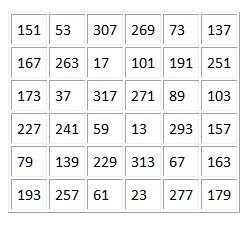Composed of distinct primes, it is both pandiagonal and symmetrical.990 is the best possible VantageScore credit rating.

Source: Prime Curios!

## Wednesday, May 20, 2009

### 1251

1251 = 32 x 139.

1251 is 20001 in base 5.

1251 is a mountain number, where the first and last digits are 1.

1251 is the sum of three fourth powers: 1251 = 54 + 54 + 14.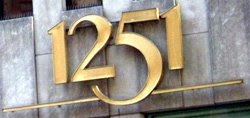The
Sharp PC-1251, produced in 1982, was a full-fledged computer in a small, calculator-like package.

## Tuesday, May 19, 2009

### 417

417 = 3 x 139.

417 is the smallest integer n such that n, n + 1, n + 2, and n + 3 all have different numbers of distinct prime factors: 417 = 3 x 139; 418 = 2 x 11 x 19; 419 is a prime; 420 = 22 x 3 x 5 x 7.

Inserting a 1 between adjacent digits of 417 produces a prime: 41117.

417 is a number that becomes prime if any digit is deleted: 17, 47, and 41.Telephone area code 417 serves southwestern Missouri.

## Friday, May 15, 2009

### 260

260 = 22 x 5 x 13.

260 has two representations as a sum of two squares: 260 = 22 + 162 = 82 + 142.

The sum of the first
260 primes minus the sum of the gaps between them is a prime number.

260 is a 11-gonal (or hendecagonal) number.

260 is the sum of two distinct powers of 2: 260 = 28 + 22.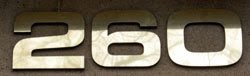260 is the number of ways that six non-attacking bishops can be placed on a four-by-four chessboard.

The number of weekdays in a year is
260, 261, or 262.

Source:
Prime Curios!

## Thursday, May 14, 2009

### 15

15 = 3 x 5.

15 is a triangular number, the sum of the first five positive integers: 1 + 2 + 3 + 4 + 5 = 15.

7 can be partitioned in
15 ways.

A three-by-three
magic square has the magic constant 15 (the sum of each row, column, or diagonal).

For
n greater than 15, at least one number between n and 2n is the product of three different primes.

15 has a unique representation as a sum of four squares: 15 = 12 + 12 + 22 + 32.

15 is the smallest number that has three representations as a sum of three primes: 15 = 2 + 2 + 11= 3 + 5 + 7 = 5 + 5 + 5.15 is the atomic number of the element phosphorus.

## Wednesday, May 13, 2009

### 349

349 is a prime number.

349 is the sum of three consecutive primes: 109 + 113 + 127 = 349.

347 and 349 form a twin prime pair.

349 has a representation as a sum of two squares: 349 = 52 + 182.

349 is the hypotenuse of a primitive Pythagorean triple: 3492 = 1802 + 2992.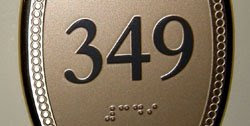The number of seats in the Swedish parliament is
349.

Source:
Wikipedia

## Tuesday, May 12, 2009

### 163

163 is a prime number.

163 is the smallest three-digit number whose square (26569) and cube (4330747) use different digits.

163 is conjectured to be the largest prime that can be represented uniquely as the sum of three squares (if squares are allowed to be zero): 163 = 12 + 92 + 92.

163 has a unique representation as a sum of three squares: 163 = 12 + 92 + 92.163 is the smallest score impossible to make using up to but not more than three darts.

Source:
Prime Curios!

## Monday, May 11, 2009

### 30

30 = 2 x 3 x 5.

30 is the smallest number with three distinct prime divisors.

30 has a unique representation as a sum of three squares: 30 = 12 + 22 + 52.

30 is a pyramidal number (the sum of all squares of integers from 1 to 4): 30 = 12 + 22 + 32 + 42.

30 is 33 in base 9.A dodecahedron is a Platonic solid with 30 edges.Source:
Number Gossip

## Friday, May 8, 2009

### 73

73 is a prime number.

73 is the least number of sixth powers needed to represent every possible integer.

73 is the smallest number (besides 1) that is one less than twice its reverse: 2 x 37 – 1 = 73.

73 is the alphanumeric value of the word NUMBER: 14 + 21 + 13 + 2 + 5 + 18 = 73.Pi Day occurs on the 73rd day of the year (March 14), except during leap years.

Source: Number Gossip

## Thursday, May 7, 2009

### 420

420 = 22 x 3 x 5 x 7.

420 is the smallest number divisible by 1 through 7.

420 is the sum of four consecutive primes: 101 + 103 + 107 + 109 = 420.

420 is the smallest argument for which Euler’s prime generating polynomial p(n) = n2 + n + 41 has more than two prime factors: p(420) = 176861 = 71 x 53 x 47. For all smaller arguments, p(n) is either prime or semiprime.In sailing, the 420 (dinghy) is a class of doublehanded racing sailboats.

Source: Prime Curios!

## Wednesday, May 6, 2009

### 1306

1306 = 2 x 653.

1306 has a representation as a sum of two squares: 1306 = 92 + 352.

1306 is the smallest four-digit number that is the sum of its digits to progressive powers: 1306 = 11 + 32 + 03 + 64.

1306 has the representation 64 + 10.In the year
1306, Robert the Bruce was crowned King of Scotland.

Source:
Number Gossip

## Tuesday, May 5, 2009

### 634

634 = 2 x 317.

634 is a Smith number. The sum of its digits equals the sum of the digits appearing in its prime divisors: 6 + 3 + 4 = 2 + 3 + 1 + 7 = 13.

The fifth root of 634 has a decimal part that begins with the digits 634.

634 has a representation as a sum of two squares: 634 = 32 + 252.634 Ute is an asteroid discovered in 1907.

## Monday, May 4, 2009

### 116

116 = 22 x 29.

116! + 1 is a prime number.

116 has a representation as a sum of two squares: 116 = 42 + 102.

116 is a composite number that when added to the sum of its prime factors becomes prime: 116 + 2 + 2 + 29 = 149.The
Hundred Years' War between France and England lasted 116 years, with periodic truces, from 1337 to 1453.

Source:
Prime Curios!

## Friday, May 1, 2009

### 28

28 = 22 x 7.

28 is the second perfect number. It is the only even perfect number of the form x3 + 1.

28 is the sum of the first five prime numbers: 2 + 3 + 5 + 7 + 11 = 28.

28 is the seventh triangular number.

28 is the smallest number with three representations as a sum of four squares: 28 = 12 + 12 + 12 + 52 = 12 + 32 + 32 + 32 = 22 + 22 + 22 + 42.

28 is a number that cannot be written as a sum of three squares.

28 is 44 in base 6.A standard domino set contains
28 dominoes.

Source:
Number Gossip